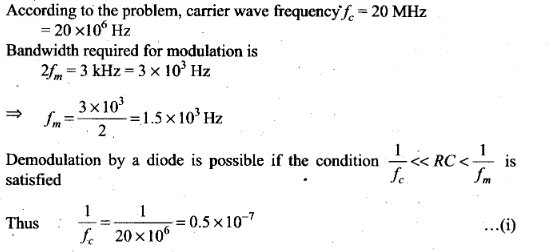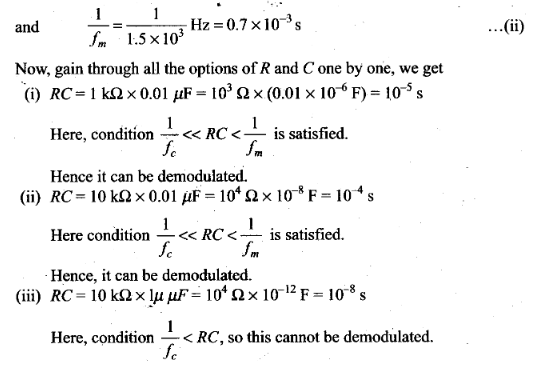# Communication Systems NCERT Exemplar Problems Solutions Physics

0
1672## NCERT Exemplar Problems Class 12 Physics Chapter 15 Communication Systems

Multiple Choice Questions (MCQs)

Question 1. Three waves A, B and C of frequencies 1600 kHz, 5 MHz and 60 MHz respectively are to be transmitted from one place to another. Which of the following is the most appropriate mode of communication?
(a) A is transmitted via space wave while B and C are transmitted via sky wave
(b) A is transmitted via ground wave, B via sky wave and C via space wave
(c) B and C are transmitted via ground wave while A is transmitted via sky wave
(d) B is transmitted via ground wave while A and C are transmitted via space wave
Solution: (b)
Key concept: The radio waves emitted from a transmitter antenna can reach the receiver antenna by the following mode of operation.
• Ground wave propagation
• Sky wave propagation
• Space wave propagation
Mode of communication frequency range:
• Ground wave propagation— 500 kHz to 1710 kHz
• Sky wave propagation — 2 MHz to 40 MHz
• Space wave propagation— 54 MHz to 42 GHz
So, A is transmitted via ground wave, B via sky wave and C via space wave.

Question 2. A loom long antenna is mounted on a 500 m tall building. The complex can become a transmission tower for waves with λ.
(a) ~400m (b) -25 m (c) -150 m (d) -2400 m
Solution: (a) Length of the building (l) is
l = 500 m
and length of antenna = 100 m
and we know, wavelength of the wave which can be transmitted by
L =λ/4. So, λ~ 4l= 4 x 100 = 400 m
Wavelength (λ) is nearly equal to 400 m.

Question 3. A1 kW signal is transmitted using a communication channel which provides attenuation at the rate of -2dB per km. If the communication channel has a total length of 5 km, the power of the signal received is [gain in dB =10 log10(p0/pi)]
(a) 900 W (b) 100 W (c) 990 W (d) 1010 W
Solution: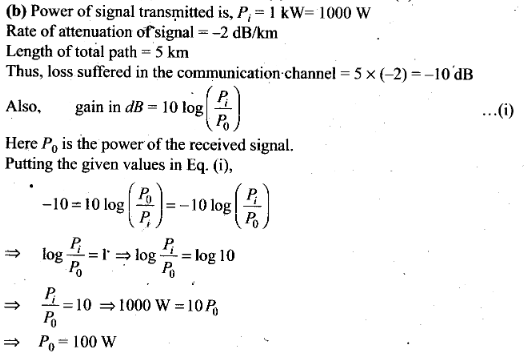Question 4. A speech signal of 3 kHz is used to modulate a carrier signal of frequency 1 MHz, using amplitude modulation. The frequencies of the side bands will be
(a) 1.003 MHz and 0.997 MHz (b) 3001 kHz and 2997 kHz
(c) 1003 kHz and 1000 kHz (d) 1 MHz and 0.997 MHz
Solution: (a)
Key concept: The process of changing the amplitude of a carrier wave in accordance with the amplitude of the audio frequency (AF) signal is known as amplitude modulation (AM).
In AM, frequency of the carrier wave remains unchanged.
Side band frequencies: The AM wave contains three frequencies fc, (fc + fm) and (fc -fm),fc is called carrier frequency, (fc +fm) and (fc -fm) are called side band frequencies.
(fc +fm): Upper side band (USB) frequency
(fc -fm): Lower side band (LSB) frequency
Side band frequencies are generally close to the carrier frequency.
According to the problem, frequency of carrier signal is fc = 1 MHz and frequency of speech signal = 3 kHz
= 3 x 10-3 MHz
= 0.003 MHz
We know that, Frequencies of side bands = (fc ± fm) = (1 + 0.003) and (1 – 0.003)
So, side band frequencies are 1.003 MHz and 0.997 MHz.

Question 5. A message signal of frequency ωm is superposed on a carrier wave of frequency ωc to get an Amplitude Modulated Wave (AM). The frequency of the AM wave will be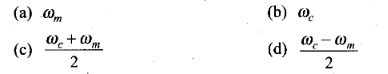Solution: (b)
Key concept: The process of changing the amplitude of a carrier wave in accordance with the amplitude of the audio frequency (AF) signal is known as amplitude modulation (AM).
In AM, frequency of the carrier wave remains unchanged or we can say that the frequency of modulated wave is equal to the frequency of carrier wave. Now, according to the problem, frequency of carrier wave is fc.
Thus the amplitude modulated wave also has frequency fc.

Question 6. I-V Characteristics of 4 devices are shown in figure.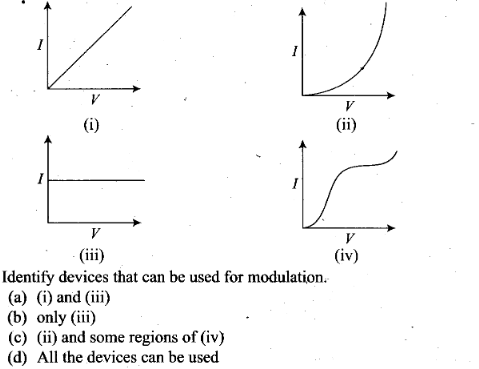Solution: Key concept: A square law modulator is the device which can produce modulated waves by the application of the message signal and the carrier wave.
Square law modulator is used for modulation purpose. Characteristics shown by (i) and (iii) correspond to linear devices.
And by (ii) corresponds to square law device which shows non-linear relations. Some part of (iv) also follow square law.
Hence, (ii) and (iv) can be used for modulation.

Question 7. A male voice after modulation-transmission sounds like that of a female to the receiver. The problem is due to
(a) poor selection of modulation index (selected 0 < m <1)
(b) poor bandwidth selection of amplifiers
(c) poor selection of carrier frequency
(d) loss of energy in transmission.
Solution: (b) In this problem, the frequency of modulated signal received becomes more, due to improper selection of bandwidth.
This happens because bandwidth in amplitude modulation is equal to twice the frequency of modulating signal.
But, the frequency of male voice is less than that of a female.

Question 8. A basic communication system consists of
A. transmitter.
B. information source.
C. user of information.
D. channel.
Choose the correct sequence in which these are arranged in a basic communication system.
Solution: (b) A basic communication system consists of an information source, a transmitter, a link (channel) and a receiver or a communication system is the set-up used in the transmission and reception of information from one place to another.
The whole system consist of several elements in a sequence. It can be represented as the diagram given below: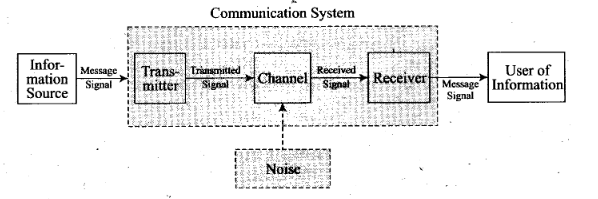Question 9. Identify the mathematical expression for amplitude modulated wave,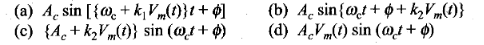Solution: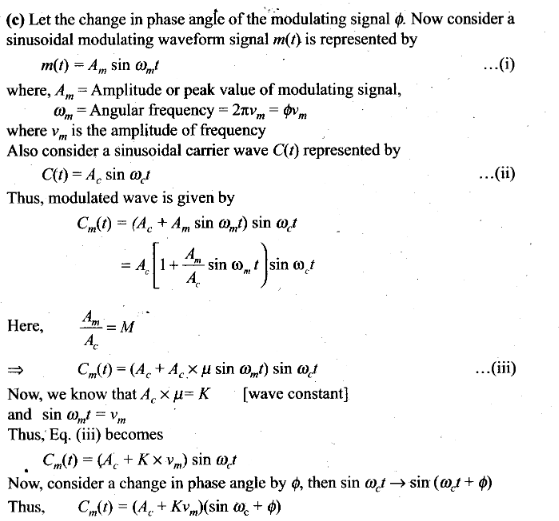One or More Than One Correct Answer Type

Question 10. An audio signal of 15 kHz frequency cannot be transmitted over long distances without modulation, because
(a) the size of the required antenna would be at least 5 km which is not convenient
(b) the audio signal cannot be transmitted through sky waves
(c) the size of the required antenna would be at least 20 km, which is not convenient
(d) effective power transmitted would be very low, if the size of the antenna is less than 5 km
Solution: (a, b, d)
Key concept: Size of the antenna or aerial. For transmitting a signal, we need an antenna or an aerial. This antenna should have a size comparable to the wavelength of the signal (at least 1/4 in dimension) so that the antenna properly senses the time variation of the signal. For an electromagnetic wave of frequency 20 kHz, the wavelength λ is 15 km. Obviously, such a long antenna is not possible to construct and operate. Hence direct transmission of such baseband signals is not practical. We can obtain transmission with reasonable antenna s if transmission frequency is high (for example,
if n is 1 MHz, then λ is 300 m). Therefore, there is a need of translating the information contained in our original low frequency baseband signal into high or radio frequencies before transmission.
Effective power radiated by an antenna: A theoretical study of radiation from a linear antenna (length l) shows that the power radiated is proportional to (1/λ)2 . This implies that for the same antenna length, the power radiated increases with decreasing λ, i.e., increasing frequency. Hence, the effective power radiated by a long wavelength baseband signal would be small. For a good transmission,we need high powers and hence this also points out to the need of using high frequency transmission.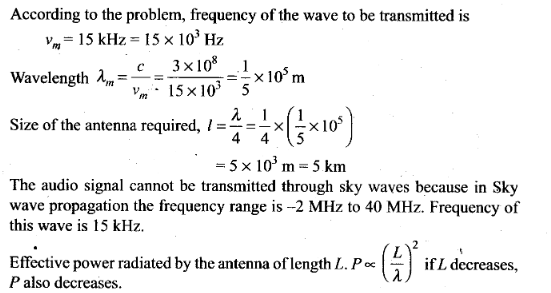Question 11. Audio sine waves of 3 kHz frequency are used to amplitude modulate a carrier signal of 1.5 MHz. Which of the following statements are true?
(a) The side band frequencies are 1506 kHz and 1494 kHz
(b) The bandwidth required for amplitude modulation is 6 kHz
(c) The bandwidth required for amplitude modulation is 3 MHz
(d) The side band frequencies are 1503 kHz and 1497 kHz
Solution: (b, d)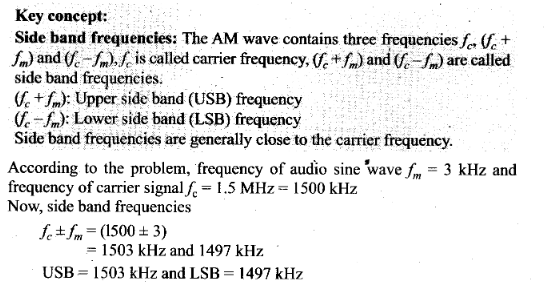Also,bandwidth =2 fm=2 x 3=6 KHz

Question 12. A TV transmission tower has a height of 240 m. Signals broadcast from this tower will be received by LOS communication at a distance of (assume the radius of earth to be (6.4 x 106 m)
(a) 100 km (b) 24 km (c) 55 km (d) 50 km
Solution: (b, c, d)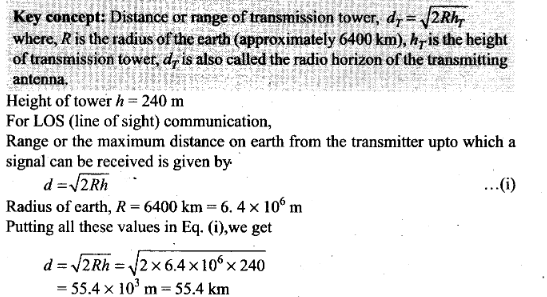Therefore, the range of 55.4 km covers the distance 24 km, 55 km and 50 km.

Question 13. The frequency response curve (figure) for the filter circuit used for production of AM wave should be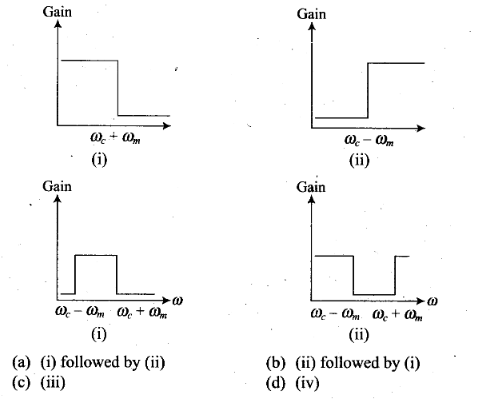Solution:(a, b, c)
Key concept:
(i) Side band frequencies-. The AM wave contains three frequencies fc ,(fc +fm) and (fc-fm),fc is called carrier frequency, (fc +fm) and (fc -fm) are called side band frequencies.
(fc + fm)- Upper side band (USB) frequency
(fc – fm): Lower side band (LSB) frequency
Side band frequencies are generally close to the carrier frequency,
(ii) Bandwidth: The two side bands lie on either side of the carrier frequency at equal frequency interval ωm.
So, bandwidth = {(ωc + ωm) – (ωc – ωm)} = 2ωm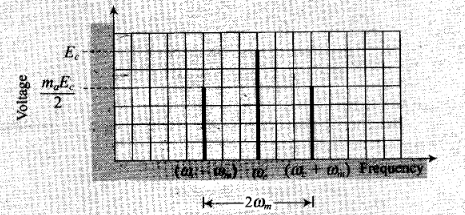To produce an amplitude modulated wave, bandwidth is given by the difference between upper side band frequency and lower side band frequency. Bandwidth = ωUSB – ωLSB = (ωc + ωm) – (ωc – ωm)

Question 14. In amplitude modulation, the modulation index m is kept less than or equal to 1 because
(a) m> 1, will result in interference between carrier frequency and message frequency, resulting into distortion.
(b) m > 1, will result in overlapping of both side bands resulting into loss of information
(c) m > 1, will result in change in phase between carrier signal and message signal.
(d) m > 1, indicates amplitude of message signal greater than amplitude of carrier signal resulting into distortion.
Solution: (b, d)
Key concept: Modulation index: The ratio of change of amplitude of carrier wave to the amplitude of original carrier wave Is called the modulation factor or degree of modulation or modulation index (ma).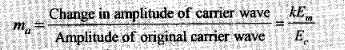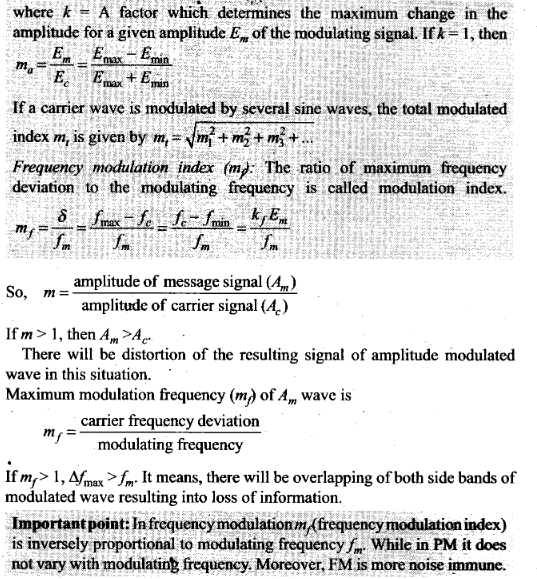Question 15. Which of the following would produce analog signals and which would produce digital signals?
(a) A vibrating tuning fork
(b) Musical sound due to a vibrating sitar string
(c) Light pulse
(d) Output of NAND gate
Solution: Analog and digital signals are the gateway of information or we can say that they are used to transmit information through electric signals. In both these signals, the information such as any audio or video is transformed into electric signals.
The difference between analog and digital technologies is that in analog technology, information is translated into electric pulses of varying amplitude. In digital technology, translation of information is into binary formal (zero or one) where each bit is representative of two distinct amplitudes. So, output of a NAND gate and a light pulse produces a digital signal.
Thus, (a) and (b) would produce analog signal and (c) and (d) would produce digital signals.

Question 16. Would sky waves be suitable for transmission of TV signals of 60 MHz frequency?
Solution: A signal to be transmitted through sky waves must have a frequency range of 1710 kHz to 40 MHz.
But, here the frequency of TV signals are 60 MHz which is beyond the required range (frequency range: there is a maximum frequency of EM waves called critical frequency, above which wave cannot reflect back).
So, sky waves will not be suitable for transmission of TV signals of 60 MHz frequency.
Important point: Sky wave propagation: These are the waves which are reflected back to the earth by ionosphere.
Ionosphere is a layer of atmosphere having charged particles, ions and electrons and extended above 80 km – 300 km from the earth’s surface.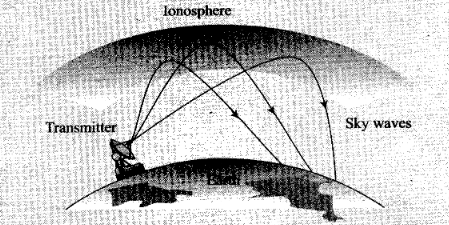Question 17. Two waves A and B of frequencies 2 MHz and 3 MHz, respectively are beamed in the same direction for communication via sky wave. Which one of these is likely to travel longer distance in the ionosphere before suffering total internal reflection?
Solution: We know that refractive index p of a layer is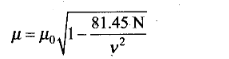The refractive index of wave B is more than refractive index of wave A because frequency of wave B is more than wave A (as refractive index increases with frequency increases).
Sin i / sin r = µ (lesser the value of r larger the value of µ )
For higher frequency wave (i.e., higher refractive index) the angle of refraction is less, i.e., bending is less. So, wave B travels longer distance in the ionosphere before suffering total internal reflection.
Importance point: Refractive index of a medium is that characteristic which decides speed of light in it.
Dependence of Refractive index:
(i) Nature of the media of incidence and refraction.
(ii) Colour of light or wavelength of light.
(iii) Temperature of the media: Refractive index decreases with the increase in temperature.
Total internal reflection: When a ray of light goes from denser to rarer medium it bends away from the normal and as the angle of incidence in denser medium increases, the angle of refraction in rarer medium also increases and at a certain angle, angle of refraction becomes 90°. This angle of incidence is called critical angle (C).
When angle of incidence exceeds the critical angle then light ray comes back into the same medium after reflection from interface. This phenomenon is called Total internal reflection (TIR).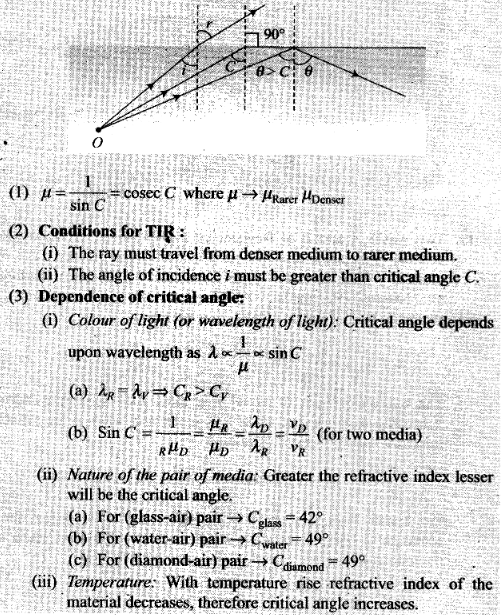Question 18. The maximum amplitude of an AM wave is found to be 15 V while its minimum amplitude is found to be 3 V. What is the modulation index?
Solution: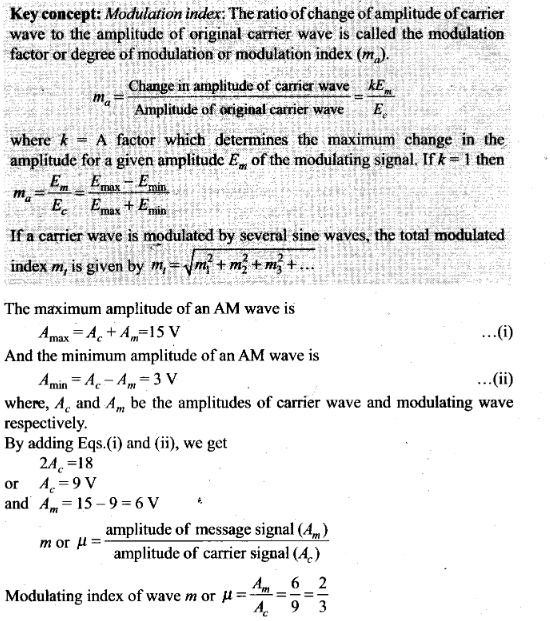Question 19. Compute the LC product of a tuned amplifier circuit required to generate a carrier wave of 1 MHz for amplitude modulation.
Solution: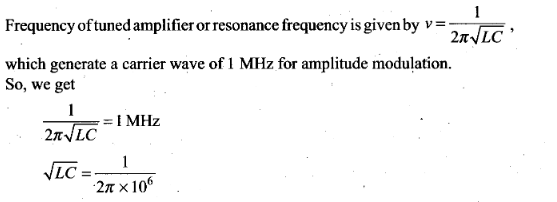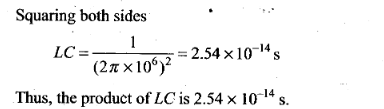Question 20. Why is an AM signal likely to be more noisy than a FM signal upon transmission through a channel?
Solution: An AM signal likely to be more noisy than FM signal through a channel because in case of AM, the instantaneous voltage of carrier waver waves is varied by the modulating wave voltage So, during the transmission, noise signals can also be added and receiver assumes noise a part of the modulating signal. In case of FM, the frequency of carrier waves is changed as the change in the instantaneous voltage of modulating waves. This can be done by mixing and not while the signal transmitting in channel. So, noise does not affect FM signal or simply we can say that noise signals are difficult to filter out in AM reception whereas FM receivers easily filter out noise.
Important point: In frequency modulation mf (frequency modulation index) is inversely proportional to modulating frequency fm. While in PM it does not vary with modulating frequency. Moreover, FM is more noise immune.

Question 21. Figure shows a communication system. What is the output power when input signal is of 1.01 mW? [Gain in dB = 10 log10 (P0 / P1)]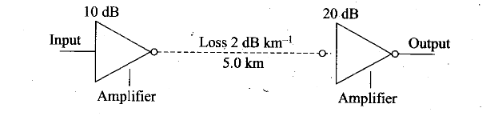Solution: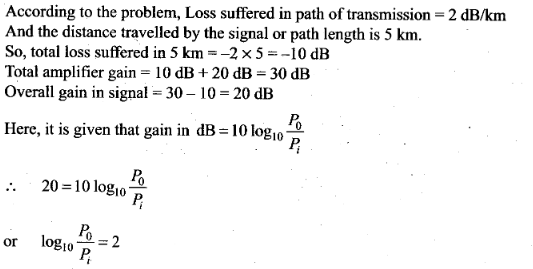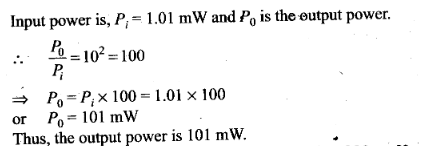Question 22. A TV transmission tower antenna is at a height of 20 m. How much service area can it cover if the receiving antenna is (i) at ground level, (ii) at a height of 25 m? Calculate the percentage increase in area covered in case (ii) relative to case (i).
Solution: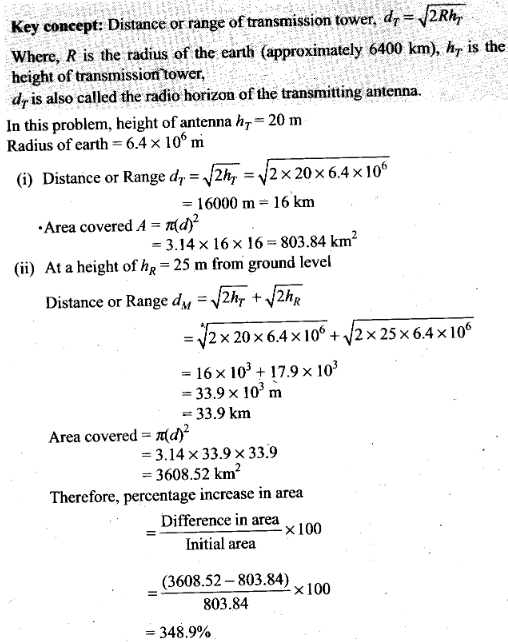Question 23. If the whole earth is to be connected by LOS communication using space waves (no restriction of antenna size or tower height), what is the minimum number of antennas required? Calculate the tower height of these antennas in terms of earth’s radius.?
Solution:
Key concept: Distance or range of transmission tower, dT =√2RhT
where, R is the radius of the earth (approximately 6400 km). hT is the height of transmission tower, .
dT is also called the radio horizon of the transmitting antenna.
Let us consider the figure given below to solve this problem.
Assume the height of transmitting antenna or receiving antenna in order to cover the entire surface of earth through communication is h1, i.e. hT = hR and radius of earth is R. If dM is the line-of-sight distance between the transmission and receiving antennas, then maximum distance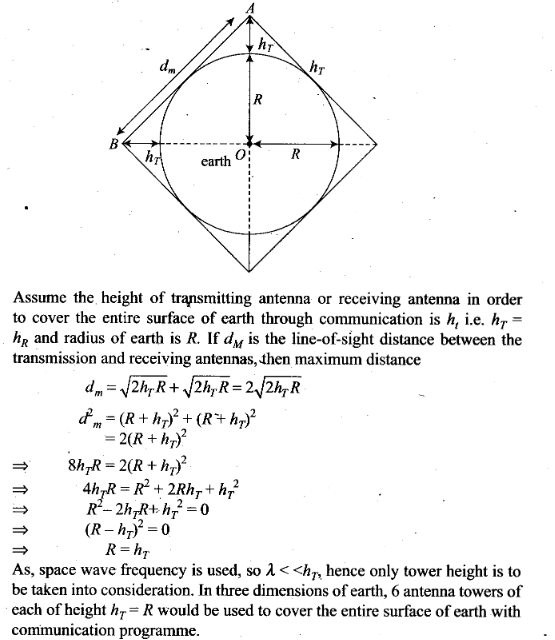Question 24. The maximum frequency for reflection of sky waves from a certain layer of the ionosphere is found to be fmax= 9(Nmax)1/2, where Nmax is the maximum electron density at that layer of the ionosphere.
On a certain day it is observed that signals of frequencies higher than 5 MHz are not received by reflection from the F1 layer of the ionosphere while signals of frequencies higher than 8 MHz are not received by reflection from the F2 layer of the ionosphere. Estimate the maximum electron densities of the F1 and F2 layers on that day.
Solution: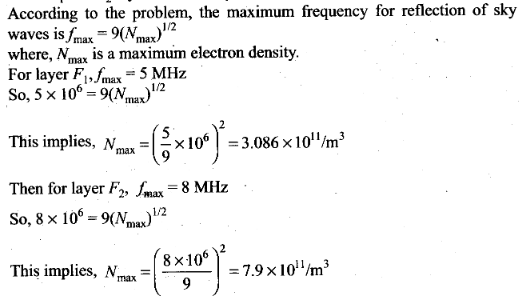Question 25. On radiating (sending out) and AM modulated signal, the total radiated power is due to energy carried by ωc, (ωc – ωm) and (ωc + ωm). Suggest ways to minimise cost of radiation without compromising on information.
Solution:
Key concept: Side band frequencies. The AM wave contains three frequencies ωc, (ωc + ωm) and (ωc – ωm), ωc is called carrier frequency, (ωc + ωm) and ( ωc – ωm) are called side band frequencies.
c + ωm) = Upper side band (USB) frequency
c – ωm) =Lower side band (LSB) frequency
Side band frequencies are generally close to the carrier frequency.
Only side band frequencies contain information in amplitude modulated signal, [only (ωc+ ωm) and (ωc + ωm)].
Here, the total radiated power is due to energy carried by ωc, (ωc – ωm) and (ωcm)
For reduction of cost of radiation without compromising on information ωc can be left and transmitting the frequencies (ωc + ωm), (ωc – ωm) or both (ωc + ωm) and (ωc – ωm).

Question 26. The intensity of a light pulse travelling along a communication channel decreases exponentially with distance x according to the relation I = I0e-ax, where I0 is the intensity at x = 0 and α is the attenuation constant.
(a) Show that the intensity reduces by 75% after a distance of (In4/α).
(b) Attenuation of a signal can be expressed in decibel (dB) according to the relation dB=10 log10(I/I0).What is the attenuation in dB/km for an optical fibre in which the intensity falls by 50% over a distance of 50 km?
Solution: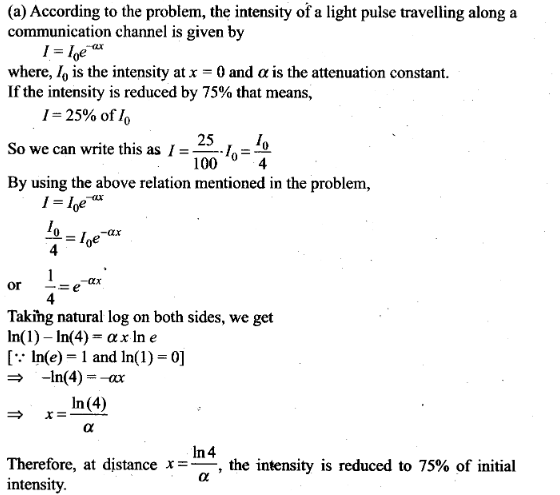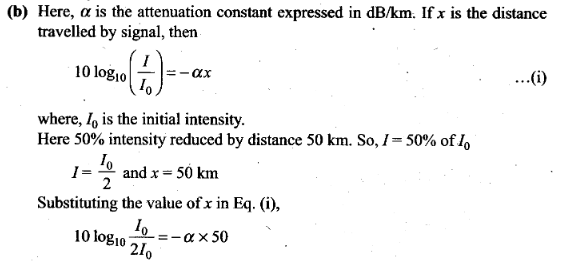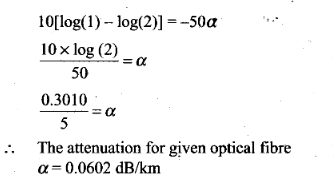Question 27. A 50 MHz sky wave takes 4.04 ms to reach a receiver via re-transmission from a satellite 600 km above Earth’s surface. Assuming re-transmission time by satellite negligible, find the distance between source and receiver. If communication between the two was to be done by Line of Sight (LOS) method, what should size and placement of receiving and transmitting antenna be?
Solution: Let the receiver is at point A and source is at B.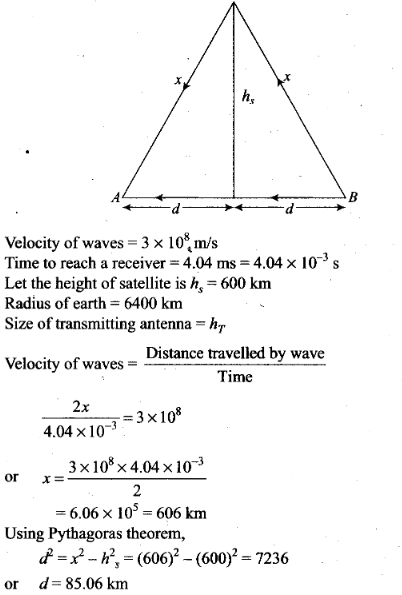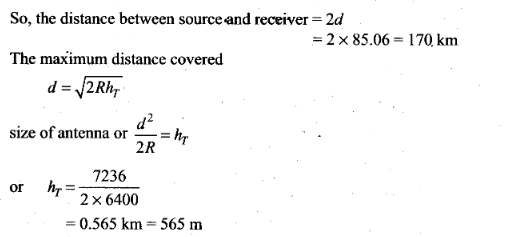Question 28. An amplitude modulated wave is as shown in figure. Calculate
(i) the percentage modulation,
(ii) peak carrier voltage and
(iii) peak value of information voltage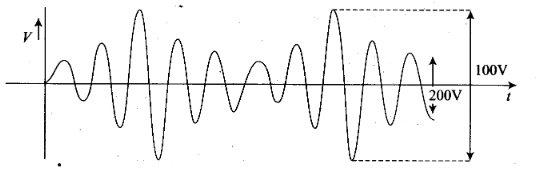Solution: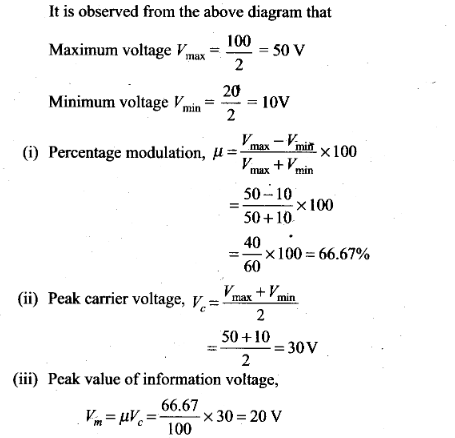Question 29. (i) Draw the plot of amplitude versus ω for an amplitude modulated wave whose carrier wave (ω > ωc) is carrying two modulating signals, ω1 and ω2 2> ω1).
(ii) Is the plot symmetrical about ωc? Comment especially about plot in region (ω < ωc ).
(iii) Extrapolate and predict the problems one can expect if more waves are to be modulated.
(iv) Suggest solutions to the above problem. In the process can one understand another advantage of modulation in terms of bandwidth?
Solution: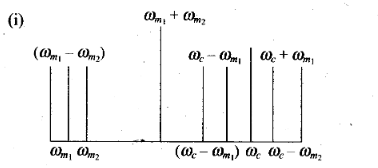(ii) In the plotted graph shown, we note that frequency spectrum is not symmetrical about ωc. Crowding of spectrum is present for ω < ωc.
(iii) If more modulating signals are present then there will be more crowding in the modulation signal in the region ω <ωc. That will result more chances of mixing of signal.
(iv) To accommodate more signals, we should increase bandwidth and frequency carrier waves ωc. This shows that large carrier frequency
enables to carry more information (i.e., more ωm) and the same will in turn increase bandwidth.

Question 30. An audio signal is modulated by a carrier wave of 20 MHz such that the bandwidth required for modulation is 3 kHz. Could this wave be demodulated by a diode detector which has the values of R and C as
(i) R = 1 kΩ, C= 0.01 µF?
(ii) R= 10 kΩ, C=0.01 µF?
(iii) R = 10 kΩ, C = 0.1 µF?
Solution: